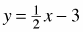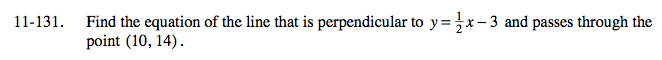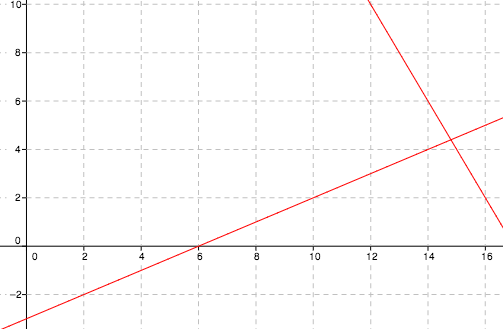Home > A2C > Chapter 11 > Lesson 11.3.1 > Problem11-131

11-131.

Find the equation of the line that is perpendicular toand passes through the point (10, 14). Homework Help ✎A perpendicular line has a slope that is the opposite reciprocal of the slope of the given line.

Using y = mx + b, substitute the m, y, and x. Now solve for b.

y = −2x + 34
The lines are shown to the right.